Uniwersytet w Białymstoku - Centralny System Uwierzytelniania Nie jesteś zalogowany | zaloguj się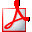Drukuj sylabus

# Partial Differential Equations

## Informacje ogólne

 Kod przedmiotu: 360-MS2-2RRC2a Kod Erasmus / ISCED: 11.105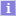/ (0541) MatematykaNazwa przedmiotu: Partial Differential Equations Jednostka: Wydział Matematyki Grupy: Punkty ECTS i inne: 5.00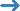zobacz reguły punktacji Język prowadzenia: angielski Rodzaj przedmiotu: obowiązkowe Założenia (opisowo): (tylko po angielsku) Student should know basic methods from one-dimensional and multidimensional mathematical analysis and ordinary differential equations. Skrócony opis: (tylko po angielsku) Course profile: academic Form of study: stationary Academic discipline: Mathematics, field of study in science: mathematics Prerequisities: Mathematical Analysis, Ordinary Differential Equations lecture 30 h. exercise class 30 h. ECTS credits: 5 Pełny opis: (tylko po angielsku) Course is devoted to classical theory of partial differential equations. After course students should know basic, classical methods of solving the first and the second order PDEs, especcialy equations of mathematical physics such as heat equation, wave equation and Laplace equation. Course objectives: Knowledge of fundamenta notions and theorems: 1. Cauchy-Kowalewska theorem. 2. Integration of first order quasi-linear and linear PDEs. First integrals. Hamiltonian systems. 3. Classification of second order PDEs. 4. Boundary value problems of different kinds. Well-posed boundary value problems. 5. Hiperbolic equations . Cauchy problem for wave equations. mixed boundary problem for wave equation. 6. Elliptic equations. Properties of harmonic functions. Green function and its properties. Solution of Dirichlet problem. 7. Parabolic equations. Heat equation. Maximum and minimum principles. existence and uniqueness theorem for solutions of Cauchy problem for heat equation. 8. Applications of knowledge to solving theoretical problems as well as to practical ones. Verification methods: lectures, exercises, consultations, studying literature, home works, discussions in groups. Balance of student workload: attending lectures15x2h = 30h attending exercise classes 15x2h = 30h preparation for classes 7x3h = 21h completing notes after exercises and lectures 7x2h = 14h consultations 12x1h = 12h the final examination: preparation.and take 12h + 3h = 15h control works: repeating the material and preparation 3x4h = 12h Quantitative description Direct interaction with the teacher: 75 h., 3 ECTS Practical exercises: 77 h., 3 ECTS Literatura: (tylko po angielsku) 1. L. Evans Partial Differential Equations, Springer 1998 2. M. Renardy, R.C.Rogers An introduction to partial differential equations, Springer 1993 3. E.C. Zachmanoglou, D. Thoe Introduction to partial differential equations with applications 1967 4. P. Olver Introduction to partial differential equations, Springer 2013 Efekty uczenia się: (tylko po angielsku) Learning outcomes: Student knows classification of first order partial differential equations (PDE), understands theorem of existence and uniqueness of solutions of Cauchy problem for quasilinear first order PDE. Student knows a notion of first integral; is able to construct general solution of problem for quasilinear first order PDEs by using characteristics. KA7_WG02, KA7_WG03, KA7_UW02, KA7_UW06 Student knows classification of first order PDEs and boudary value problems of different kinds; knows a notion of well-posed problem for mathematical physics equations and understands connection between equations and physical processes, described by them. Student is able to determine the type of PDE with two independent variables.KA7_WG02, KA7_WG04, KA7_WG06, KA7_UW06, KA7_UW10 Student knows a canonical form of hiperbolic PDE, methods of wave propagation, d'Alembert formula and understand Kirchoff formula. Student is able to use these formulas in simple examples. KA7_WG02, KA7_WG06, KA7_UW06 Student knows fundamental solution of Laplace equation, properties of harmonic functions, notion of Green function and its applications.KA7_WG02, KA7_WG03, KA7_UW01, KA7_UW06 Student understands maximum pronciple and uniqueness of solution in boundary value problem for heat equation with two independent variables. Student knows fundamental solution and formula for solutions in Cauchy problem for heat equation.KA7_WG02, KA7_WG04, KA7_WG05, KA7_UW06, KA7_UW10 Student obtains basic practice in creative development of theory of differential equations. KA7_KK01, KA7_KK02, KA7_KK07, KA7_UU01 Metody i kryteria oceniania: (tylko po angielsku) The overall form of credit for the course: final exam

## Zajęcia w cyklu "Rok akademicki 2022/23" (jeszcze nie rozpoczęty)

 Okres: 2022-10-01 - 2023-06-30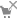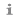Wybrany podział planu: ten tydzień cykl przedmiotuzobacz plan zajęć Typ zajęć: Ćwiczenia, 30 godzinwięcej informacji Wykład, 30 godzinwięcej informacji Koordynatorzy: Tomasz Czyżycki, Aneta Sliżewska Prowadzący grup: (brak danych) Lista studentów: (nie masz dostępu) Zaliczenie: Egzamin Pełny opis: (tylko po angielsku) Course objectives: Knowledge of fundamenta notions and theorems: 1. Cauchy-Kowalewska theorem. 2. Integration of first order quasi-linear and linear PDEs. First integrals. Hamiltonian systems. 3. Classification of second order PDEs. 4. Boundary value problems of different kinds. Well-posed boundary value problems. 5. Hiperbolic equations . Cauchy problem for wave equations. mixed boundary problem for wave equation. 6. Elliptic equations. Properties of harmonic functions. Green function and its properties. Solution of Dirichlet problem. 7. Parabolic equations. Heat equation. Maximum and minimum principles. existence and uniqueness theorem for solutions of Cauchy problem for heat equation. 8. Applications of knowledge to solving theoretical problems as well as to practical ones. Verification methods: lectures, exercises, consultations, studying literature, home works, discussions in groups. Literatura: (tylko po angielsku) 1. L. Evans Partial Differential Equations, Springer 1998 2. M. Renardy, R.C.Rogers An introduction to partial differential equations, Springer 1993 3. E.C. Zachmanoglou, D. Thoe Introduction to partial differential equations with applications 1967 4. P. Olver Introduction to partial differential equations, Springer 2013
Opisy przedmiotów w USOS i USOSweb są chronione prawem autorskim.
Właścicielem praw autorskich jest Uniwersytet w Białymstoku.